## Getting Started: OPTMODEL Procedure

Optimization or mathematical programming is a search for a maximum or minimum of an objective function (also called a cost function), where search variables are restricted to particular constraints. Constraints are said to define a feasible region (see Figure 5.1).

Figure 5.1: Examples of Feasible Regions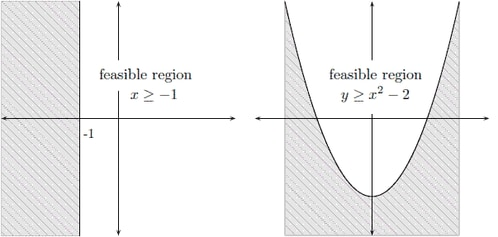A more rigorous general formulation of such problems is as follows.

Let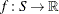be a real-valued function. Find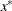such that

•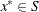•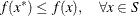Note that the formulation is for the minimum of
and that the maximum ofis simply the negation of the minimum of
.

Here, functionis the objective function, and the variable in the objective function is called the optimization variable (or decision variable).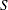is the feasible region. Typicallyis a subset of the Euclidean space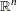specified by the set of constraints, which are often a set of equalities () or inequalities (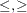) that every element inis required to satisfy simultaneously. For the special case where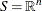, the problem is an unconstrained optimization. An elementofis called a feasible solution to the optimization problem, and the value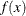is called the objective value. A feasible solutionthat minimizes the objective function is called an optimal solution to the optimization problem, and the corresponding objective value is called the optimal value.

In mathematics, special notation is used to denote an optimization problem. Generally, you can write an optimization problem as follows: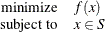Normally, an empty body of constraint (the part after subject to) implies that the optimization is unconstrained (that is, the feasible region is the whole space). The optimal solution () is denoted as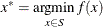The optimal value (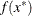) is denoted as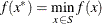Optimization problems can be classified by the forms (linear, quadratic, nonlinear, and so on) of the functions in the objective and constraints. For example, a problem is said to be linearly constrained if the functions in the constraints are linear. A linear programming problem is a linearly constrained problem with a linear objective function. A nonlinear programming problem occurs where some function in the objective or constraints is nonlinear, and so on.